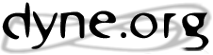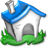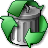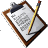:: Re: [Frei0r] Plugin documentation?CatalàDanskDeutschΕλληνικάEnglishEspañolsuomiFrançaismagyarItaliano日本語NederlandsPolskiPortuguêsPortuguês BrasileiroAuthor: James Harkins
Date:
To: frei0r@lists.dyne.org
Subject: Re: [Frei0r] Plugin documentation?
On Sun, 23 Aug 2020 17:11:27 +0800
‪‪jamshark70@???‬‬ <jamshark70@???> wrote:

> The catch is, I don't know what plugins are there (beyond the
> directory listing of .so files) or what the parameters are.

I ended up finding one place to start... kdenlive uses XML files to
describe effects, and it refers to a bunch of frei0r plug-ins. So I
wrote a dumb little program to scan
'/usr/share/kdenlive/effects/frei0r*.xml' and print out names,
descriptions and parameters, e.g.

Cartoon (frei0r.cartoon): Cartoonify video, do a form of edge detect
- triplevel (constant) = 500 (0 - 100000)
- diffspace (constant) = 1 (0 - 256)

I'll attach the file to use or discard as you like. Maybe it's useful
to some other people.

hjh
Alpha operations (frei0r.alpha0ps): Display and manipulation of the alpha channel
- Display (list) = Image (Image, Alpha as gray, Gray + red, Selection on black, Selection on gray, Selection on white, Selection on checkers)
- Display input alpha (bool) = 0
- Operation (list) = NO OP (NO OP, Shave, Shrink hard, Shrink soft, Grow hard, Grow soft, Threshold)
- Threshold (simplekeyframe) = 500
- Shrink/grow amount (simplekeyframe) = 500
- Invert (bool) = 0

Alpha operations (frei0r.alpha0ps): Display and manipulation of the alpha channel
- Display (list) = Image (Image, Alpha as gray, Gray + red, Selection on black, Selection on gray, Selection on white, Selection on checkers)
- Display input alpha (bool) = 0
- Operation (list) = NO OP (NO OP, Shave, Shrink hard, Shrink soft, Grow hard, Grow soft, Threshold, Blur)
- Threshold (simplekeyframe) = 500
- Shrink/Grow/Blur amount (simplekeyframe) = 500
- Invert (bool) = 0

- Position (simplekeyframe) = 500
- Transition width (simplekeyframe) = 500
- Tilt (simplekeyframe) = 500
- Min (simplekeyframe) = 0
- Max (simplekeyframe) = 1000
- Operation (list) = Write on clear (Write on clear, Max, Min, Add, Subtract)

Alpha shapes (frei0r.alphaspot): Draws simple shapes into the alpha channel
- Shape (list) = Rectangle (Rectangle, Ellipse, Triangle, Diamond)
- Position X (simplekeyframe) = 500
- Position Y (simplekeyframe) = 500
- Size X (simplekeyframe) = 100
- Size Y (simplekeyframe) = 100
- Tilt (simplekeyframe) = 500
- Transition width (simplekeyframe) = 200
- Min (simplekeyframe) = 0
- Max (simplekeyframe) = 1000
- Operation (list) = Write on clear (Write on clear, Max, Min, Add, Subtract)

White Balance (frei0r.balanc0r): Adjust the white balance / color temperature
- Neutral Color (color) = 0xffffffff
- Green Tint (simplekeyframe) = 1200

White Balance (frei0r.balanc0r): Adjust the white balance / color temperature
- Neutral Color (color) = 0xffffffff
- Green Tint (simplekeyframe) = 1333

Baltan (frei0r.baltan): Delayed alpha smoothed blit of time

- Channel (list) = RGB (RGB, Red, Green, Blue, Alpha, Luma, Hue, Saturation)
- Luma formula (list) = Rec. 709 (Rec. 601, Rec. 709)

Brightness (frei0r.brightness): Adjusts the brightness of a source image
- Brightness (constant) = 500 (0 - 1000)

Corners (frei0r.c0rners): Four corners geometry engine
- Corner 1 X (simplekeyframe) = 2000
- Corner 1 Y (simplekeyframe) = 2000
- Corner 2 X (simplekeyframe) = 4000
- Corner 2 Y (simplekeyframe) = 2000
- Corner 3 X (simplekeyframe) = 4000
- Corner 3 Y (simplekeyframe) = 4000
- Corner 4 X (simplekeyframe) = 2000
- Corner 4 Y (simplekeyframe) = 4000
- Stretch X (simplekeyframe) = 1000
- Stretch Y (simplekeyframe) = 1000
- Feather Alpha (simplekeyframe) = 5
- Enable Stretch (bool) = 0
- Interpolator (list) = Bilinear (Nearest neighbor, Bilinear, Bicubic smooth, Bicubic sharp, Spline 4x4, Spline 6x6, Lanczos)
- Transparent Background (bool) = 1
- Alpha operation (list) = Write on clear (Write on clear, Maximum, Minimum, Add, Subtract)

Cartoon (frei0r.cartoon): Cartoonify video, do a form of edge detect
- triplevel (constant) = 500 (0 - 100000)
- diffspace (constant) = 1 (0 - 256)

Cartoon (frei0r.cartoon): Cartoonify video, do a form of edge detect
- triplevel (constant) = 99900 (0 - 100000)
- diffspace (constant) = 1 (0 - 256)

K-Means Clustering (frei0r.cluster): Clusters of a source image by color and spatial distance
- Num (constant) = 20 (0 - 40)
- Dist weight (constant) = 500 (0 - 1000)

White Balance (LMS space) (frei0r.colgate): Do simple color correction, in a physically meaningful
way
- Neutral Color (color) = 0x7f7f7fff
- Color Temperature (simplekeyframe) = 6500

- R (simplekeyframe) = 500
- G (simplekeyframe) = 500
- B (simplekeyframe) = 500
- Action (list) = Change gamma (Add constant, Change gamma, Multiply)
- Keep luma (bool) = 1
- Alpha controlled (bool) = 0
- Luma formula (list) = Rec. 709 (Rec. 601, Rec. 709)

Color Distance (frei0r.colordistance): Calculates the distance between the selected color and the current pixel and uses that value as new pixel value
- Color (color) = 0x000000ff

Color Effect (frei0r.colortap): Applies a pre-made color effect to image
- table (list) = nil ()

Contrast (frei0r.contrast0r): Adjusts the contrast of a source image
- Contrast (simplekeyframe) = 250

- Channel (list) = Red (Red, Green, Blue, Luma)
- Luma formula (list) = Rec. 709 (Rec. 601, Rec. 709)
- Curve point number (fixed) = 2 (2 - 5)
- Point 1 input value (fixed) = 0 (0 - 1)
- Point 1 output value (fixed) = 0 (0 - 1)
- Point 2 input value (fixed) = 1 (0 - 1)
- Point 2 output value (fixed) = 1 (0 - 1)
- Point 3 input value (fixed) = 0 (0 - 1)
- Point 3 output value (fixed) = 0 (0 - 1)
- Point 4 input value (fixed) = 0 (0 - 1)
- Point 4 output value (fixed) = 0 (0 - 1)
- Point 5 input value (fixed) = 0 (0 - 1)
- Point 5 output value (fixed) = 0 (0 - 1)
- Show curves (bool) = 0
- Graph position (list) = Bottom Right (Top Left, Top Right, Bottom Left, Bottom Right)

- Channel (list) = RGB (RGB, Red, Green, Blue, Alpha, Luma, Hue, Saturation)
- Luma formula (list) = Rec. 709 (Rec. 601, Rec. 709)
- Curve point number (fixed) = 0.2 (0.2 - 0.5)
- Point 1 input value (fixed) = 0 (0 - 1)
- Point 1 output value (fixed) = 0 (0 - 1)
- Point 2 input value (fixed) = 1 (0 - 1)
- Point 2 output value (fixed) = 1 (0 - 1)
- Point 3 input value (fixed) = 0 (0 - 1)
- Point 3 output value (fixed) = 0 (0 - 1)
- Point 4 input value (fixed) = 0 (0 - 1)
- Point 4 output value (fixed) = 0 (0 - 1)
- Point 5 input value (fixed) = 0 (0 - 1)
- Point 5 output value (fixed) = 0 (0 - 1)
- Show curves (bool) = 0
- Graph position (list) = Bottom Right (Top Left, Top Right, Bottom Left, Bottom Right)

Nikon D90 Stairstepping fix (frei0r.d90stairsteppingfix): Removes stairstepping artifacts from Nikon D90's 720p videos.

Defish (frei0r.defish0r): Non rectilinear lens mappings
- Amount (simplekeyframe) = 0
- DeFish (bool) = 1
- Type (list) = Equiarea (Equidistant, Orthographic, Equiarea, Stereographic)
- Scaling (list) = Fill (Fill, Center, Fit, Manual)
- Manual Scale (constant) = 500 (0 - 1000)
- Interpolator (list) = Bilinear (Nearest neighbor, Bilinear, Bicubic smooth, Bicubic sharp, Spline 4x4, Spline 6x6, Lanczos)
- Aspect type (list) = Square (Square, PAL DV, NTSC DV, HDV, Manual)
- Manual Aspect (constant) = 500 (0 - 1000)

delay0r (frei0r.delay0r): Video delay
- DelayTime (constant) = 0 (0 - 1000)

Delay grab (frei0r.delaygrab): Delayed frame blitting mapped on a time bitmap

Distort (frei0r.distort0r): Plasma
- Amplitude (simplekeyframe) = 0
- Frequency (simplekeyframe) = 0

Edge glow (frei0r.edgeglow): Edge glow filter
- lthresh (constant) = 0 (0 - 1000)
- lupscale (constant) = 0 (0 - 1000)
- lredscale (constant) = 0 (0 - 1000)

Equaliz0r (frei0r.equaliz0r): Equalizes the intensity histograms

Face blur (frei0r.facebl0r): Automatically detect and blur a face using OpenCV
- Search scale (simplekeyframe) = 120
- Neighbors (simplekeyframe) = 2
- Smallest (simplekeyframe) = 0
- Largest (simplekeyframe) = 500
- Recheck (constant) = 1 (1 - 1000)
- Ellipse (bool) = 0

Face detect (frei0r.facedetect): Detect faces and draw shapes on them using OpenCV
- Search scale (simplekeyframe) = 120
- Neighbors (simplekeyframe) = 2
- Smallest (simplekeyframe) = 0
- Recheck (constant) = 25 (0 - 1000)
- Shape (list) = circle (circle, ellipse, rectangle, random)
- Stroke (constant) = 0 (0 - 100)
- Alpha (constant) = 100 (0 - 100)
- Antialias (bool) = 0

Flippo (frei0r.flippo): Flipping in X and Y axis
- X axis (bool) = 1
- Y axis (bool) = 1

Glow (frei0r.glow): Creates a Glamorous Glow
- Blur (simplekeyframe) = 10

Denoiser (frei0r.hqdn3d): High quality 3D denoiser
- Spatial (simplekeyframe) = 40
- Temporal (simplekeyframe) = 60

Hue shift (frei0r.hueshift0r): Shifts the hue of a source image
- Hue (simplekeyframe) = 0

Blur (frei0r.IIRblur): Blur using 2D IIR filters (Exponential, Lowpass, Gaussian)
- Amount (simplekeyframe) = 40
- Type (list) (Exponential, Lowpass, Gaussian)
- Edge (bool) = 1

Key Spill Mop Up (frei0r.keyspillm0pup): Reduces the visibility of key color spill in chroma keying
- Key color (color) = 0x1010D0ff
- Target color (color) = 0xC87F65ff
- Mask type (list) = Color distance (Color distance, Transparency, Edge inwards, Edge outwards)
- Tolerance (constant) = 240 (0 - 1000)
- Slope (constant) = 400 (0 - 1000)
- Hue gate (constant) = 250 (0 - 1000)
- Saturation threshold (constant) = 150 (0 - 1000)
- Operation 1 (list) = De-Key (None, De-Key, Target, Desaturate, Luma adjust)
- Amount 1 (constant) = 500 (0 - 1000)
- Operation 2 (list) = None (None, De-Key, Target, Desaturate, Luma adjust)
- Amount 2 (constant) = 0 (0 - 1000)
- Show mask (bool) = 0
- Mask to Alpha (bool) = 0

Lens Correction (frei0r.lenscorrection): Allows compensation of lens distortion
- xcenter (simplekeyframe) = 500
- ycenter (simplekeyframe) = 500
- correctionnearcenter (simplekeyframe) = 500
- correctionnearedges (simplekeyframe) = 500
- brightness (simplekeyframe) = 0

LetterB0xed (frei0r.letterb0xed): Adds black borders at top and bottom for cinema look
- Border Width (constant) = 400 (0 - 1000)
- Transparency (bool) = 0

- Channel (list) = Luma (Red, Green, Blue, Luma)
- Input black level (simplekeyframe) = 0
- Input white level (simplekeyframe) = 1000
- Gamma (simplekeyframe) = 1000
- Black output (simplekeyframe) = 0
- White output (simplekeyframe) = 1000
- Show histogram (bool) = 0
- Histogram position (list) = Bottom Right (Top Left, Top Right, Bottom Left, Bottom Right)

- Channel (list) = Luma (Red, Green, Blue, Luma)
- Input black level (simplekeyframe) = 0
- Input white level (simplekeyframe) = 1000
- Gamma (simplekeyframe) = 1000
- Black output (simplekeyframe) = 0
- White output (simplekeyframe) = 1000
- Show histogram (bool) = 0
- Histogram position (list) = Bottom Right (Top Left, Top Right, Bottom Left, Bottom Right)

Light Graffiti (frei0r.lightgraffiti): Light Graffiti effect.
- thresholdBrightness (simplekeyframe) = 450
- thresholdDifference (simplekeyframe) = 80
- thresholdDiffSum (simplekeyframe) = 0
- sensitivity (simplekeyframe) = 100
- lowerOverexposure (simplekeyframe) = 0
- dim (simplekeyframe) = 0
- backgroundWeight (simplekeyframe) = 0
- longAlpha (simplekeyframe) = 0
- saturation (simplekeyframe) = 150
- statsBrightness (bool) = 0
- statsDifference (bool) = 0
- statsDiffSum (bool) = 0
- transparentBackground (bool) = 0
- nonlinearDim (bool) = 0
- reset (bool) = 0

Light Graffiti (frei0r.lightgraffiti): Light Graffiti effect.
- thresholdBrightness (simplekeyframe) = 450
- thresholdDifference (simplekeyframe) = 80
- thresholdDiffSum (simplekeyframe) = 0
- sensitivity (simplekeyframe) = 100
- lowerOverexposure (simplekeyframe) = 0
- dim (simplekeyframe) = 0
- backgroundWeight (simplekeyframe) = 0
- longAlpha (simplekeyframe) = 0
- saturation (simplekeyframe) = 150
- statsBrightness (bool) = 0
- statsDifference (bool) = 0
- statsDiffSum (bool) = 0
- transparentBackground (bool) = 0
- nonlinearDim (bool) = 0
- reset (bool) = 0

Luminance (frei0r.luminance): Creates a luminance map of the image

- Left (constant) = 0 (0 - 1000)
- Right (constant) = 0 (0 - 1000)
- Top (constant) = 0 (0 - 1000)
- Bottom (constant) = 0 (0 - 1000)
- Invert (bool) = 1
- Blur (constant) = 0 (0 - 1000)

Medians (frei0r.medians): Implements several median-type filters
- Type (list) = Square3x3 (Cross5, Square3x3, Bilevel, Diamond3x3, Square5x5, Temp3, Temp5, ArceBI, ML3D, ML3dEX, VarSize)
- Size (constant) = 5 (0 - 50)

Nervous (frei0r.nervous): Flushes frames in time in a nervous way

nosync0r (frei0r.nosync0r): Broken TV
- HSync (constant) = 0 (0 - 1000)

Pixelize (frei0r.pixeliz0r): Pixelize input image.
- BlockSizeX (simplekeyframe) = 10
- BlockSizeY (simplekeyframe) = 10

Video values (frei0r.pr0be): Measure video values
- Measurement (list) = RGB (RGB, Y'PbPr - rec. 601, Y'PbPr - rec. 709, HSV, HSL)
- X (constant) = 500 (0 - 1000)
- Y (constant) = 500 (0 - 1000)
- X size (constant) = 7 (0 - 25)
- Y size (constant) = 7 (0 - 25)
- 256 scale (bool) = 0
- Show alpha (bool) = 0
- Big window (bool) = 0

Oscilloscope (frei0r.pr0file): 2D video oscilloscope
- X (constant) = 500 (0 - 1000)
- Y (constant) = 500 (0 - 1000)
- Tilt (constant) = 500 (0 - 1000)
- Length (constant) = 650 (0 - 1000)
- Channel (list) = Y' (R, G, B, Y', Pr, Pb, Alpha)
- Marker 1 (constant) = 0 (0 - 1000)
- Marker 2 (constant) = 0 (0 - 1000)
- R trace (bool) = 1
- G trace (bool) = 1
- B trace (bool) = 1
- Y trace (bool) = 0
- Pr trace (bool) = 0
- Pb trace (bool) = 0
- Alpha trace (bool) = 0
- Display average (bool) = 1
- Display RMS (bool) = 1
- Display minimum (bool) = 0
- Display maximum (bool) = 0
- 256 scale (bool) = 0
- Color (list) = CCIR rec. 601 (CCIR rec. 601, CCIR rec. 709)
- Crosshair color (constant) = 0 (0 - 7)

Primaries (frei0r.primaries): Reduce image to primary colors
- Factor (constant) = 1 (0 - 32)

RGB Parade (frei0r.rgbparade): Display a histogram of R, G and B components of the video data

Saturation (frei0r.saturat0r): Adjusts the saturation of a source image
- Saturation (simplekeyframe) = 125

Crop, Scale and Tilt (frei0r.scale0tilt): Scales, Tilts and Crops an Image
- Clip left (constant) = 0 (0 - %width-1)
- Clip right (constant) = 0 (0 - %width-1)
- Clip top (constant) = 0 (0 - %height-1)
- Clip bottom (constant) = 0 (0 - %height-1)
- Scale X (constant) = 50 (1 - 1000)
- Scale Y (constant) = 50 (1 - 1000)
- Tilt X (constant) = %width/2 (0 - %width)
- Tilt Y (constant) = %height/2 (0 - %height)

scanline0r (frei0r.scanline0r): Interlaced black lines

Color Selection (frei0r.select0r): Color based alpha selection
- Color to select (color) = 0x00ff00ff
- Invert selection (bool) = 1
- Delta R / A / Hue (simplekeyframe) = 200
- Delta G / B / Chroma (simplekeyframe) = 200
- Delta B / I / I (simplekeyframe) = 200
- Selection subspace (list) = RGB (RGB, ABI, HCI)
- Subspace shape (list) = Ellipsoid (Box, Ellipsoid, Diamond)
- Edge mode (list) = Hard (Hard, Fat, Normal, Skinny)
- Operation (list) = Write on clear (Write on clear, Max, Min, Add, Subtract)

Color Selection (frei0r.select0r): Color based alpha selection
- Color to select (color) = 0x00ff00ff
- Invert selection (bool) = 1
- Selection subspace (list) = RGB (RGB, ABI, HCI)
- Subspace shape (list) = Ellipsoid (Box, Ellipsoid, Diamond)
- Edge mode (list) = Slope (Hard, Fat, Normal, Skinny, Slope)
- Delta R / A / Hue (simplekeyframe) = 200
- Delta G / B / Chroma (simplekeyframe) = 200
- Delta B / I / I (simplekeyframe) = 200
- Slope (simplekeyframe) = 0
- Operation (list) = Write on clear (Write on clear, Max, Min, Add, Subtract)

Sharpen (frei0r.sharpness): Unsharp masking (port from Mplayer)
- Amount (simplekeyframe) = 300
- Size (simplekeyframe) = 0

Sobel (frei0r.sobel): Sobel filter

SOP/Sat (frei0r.sopsat): Changes Slope, Offset, and Power of the color components, and the overall Saturation, according to the ASC CDL (Color Decision List).
- rSlope (simplekeyframe) = 100
- gSlope (simplekeyframe) = 100
- bSlope (simplekeyframe) = 100
- aSlope (simplekeyframe) = 100
- rOffset (simplekeyframe) = 0
- gOffset (simplekeyframe) = 0
- bOffset (simplekeyframe) = 0
- aOffset (simplekeyframe) = 0
- rPower (simplekeyframe) = 100
- gPower (simplekeyframe) = 100
- bPower (simplekeyframe) = 100
- aPower (simplekeyframe) = 100
- saturation (simplekeyframe) = 100

SOP/Sat (frei0r.sopsat): Changes Slope, Offset, and Power of the color components, and the overall Saturation, according to the ASC CDL (Color Decision List).
- rSlope (simplekeyframe) = 100
- gSlope (simplekeyframe) = 100
- bSlope (simplekeyframe) = 100
- aSlope (simplekeyframe) = 100
- rOffset (simplekeyframe) = 0
- gOffset (simplekeyframe) = 0
- bOffset (simplekeyframe) = 0
- aOffset (simplekeyframe) = 0
- rPower (simplekeyframe) = 100
- gPower (simplekeyframe) = 100
- bPower (simplekeyframe) = 100
- aPower (simplekeyframe) = 100
- saturation (simplekeyframe) = 100

Square Blur (frei0r.squareblur): Square blur
- Kernel size (simplekeyframe) = 50

TehRoxx0r (frei0r.tehRoxx0r): Something videowall-ish
- Interval (constant) = 0 (0 - 1000)

3-level Threshold (frei0r.threelay0r): Dynamic 3-level thresholding

3 point balance (frei0r.three_point_balance): Balances colors along with 3 points
- Black color (color) = 0x000000ff
- Gray color (color) = 0x808080ff
- White color (color) = 0xffffffff
- Split preview (bool) = 0
- Source image on left side (bool) = 1

Threshold (frei0r.threshold0r): Thresholds a source image
- Threshold (simplekeyframe) = 200

Timeout indicator (frei0r.timeout): nil
- color (color) = 0x6893C8ff
- time (simplekeyframe) = 0
- transparency (simplekeyframe) = 0

Tint (frei0r.tint0r): Maps source image luminance between two colors specified
- Map black to (color) = 0x000000ff
- Map white to (color) = 0x80FF80ff
- Tint amount (simplekeyframe) = 250

Binarize dynamically (frei0r.twolay0r): Dynamic thresholding

Vectorscope (frei0r.vectorscope): Display a vectorscope of the video data

Vertigo (frei0r.vertigo): Alpha blending with zoomed and rotated images
- PhaseIncrement (simplekeyframe) = 2
- Zoomrate (simplekeyframe) = 101

Vertigo (frei0r.vertigo): Alpha blending with zoomed and rotated images
- PhaseIncrement (simplekeyframe) = 2
- Zoomrate (simplekeyframe) = 101

Vignette (frei0r.vignette): Natural lens vignetting effect
- aspect (simplekeyframe) = 500
- clearCenter (simplekeyframe) = 0
- soft (simplekeyframe) = 600